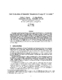## Fast Evaluation of Ensemble Transients of Large IP Networks##### Files
CS-TR-3903.ps(700.76 KB)
We extend a numerical approximate solution method (the Z-iteration) to time-dependent open networks of M(t)/M(t)/1/$\infty$ and M(t)/M(t)/1/K queues, and apply the method to obtain transient performance metrics of large IP networks. The method generates a set of coupled differential equations, one for each queue in the network. The equations are numerically unstable under certain conditions (e.g., large bandwidths and buffers), and we present techniques to overcome this problem. The resulting numerical procedure is accurate and very fast. For example, a 20-second evolution for a 1000-node network with high-speed links ($\approx 10^4$packets/sec) and large buffers ($\approx 10^4$packets) was obtained in 18 minutes on an Ultra Sparc, whereas simulation would take days.